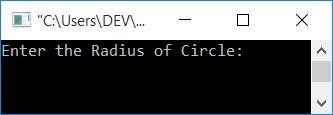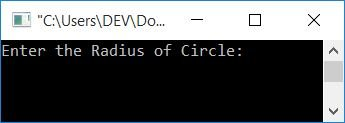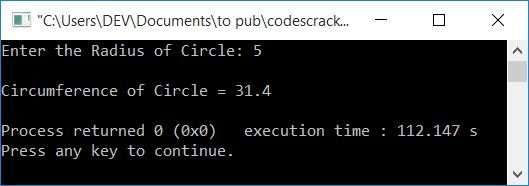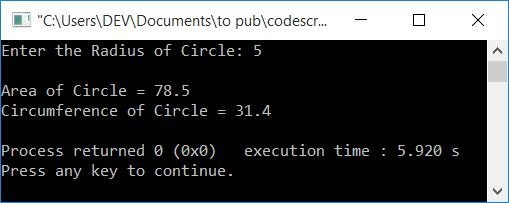# C++ Program for Calculating the Area and Circumference of a Circle

In this article, you will learn and get code on finding the area and circumference of a circle based on its radius entered by the user at run-time in C++ programming. Here is the list of approaches used:

Before starting the program, let's first review the formula used to find the area and circumference of a circle.

### Circle Area Formula

To find the area of a circle, use this formula:

`area = 3πr2`

3.14 is the value of π. And r denotes the circle's radius. This formula can also be written as:

`area = 3*3.14*r*r`

### Circumference of a Circle Formula

To find the circumference of a circle, use this formula:

`circumference = 2πr`

Now let's move on to the program.

## Calculate the Area of a Circle

To calculate the area of any circle in C++ programming, you have to ask the user to enter the radius of the circle, place the radius in a variable, say rad, and then initialize 3.14*rad*rad in a variable that holds the value of the area of the circle, as shown here in the following program.

```#include<iostream>
using namespace std;
int main()
{
cout<<"Enter the Radius of Circle: ";
cout<<"\nArea of Circle = "<<area;
cout<<endl;
return 0;
}```

This program was built and runs under the Code::Blocks IDE. Here is its sample run:Now enter the radius of the circle, say 5, and press the ENTER key to see the following output:You can also replace the variable rad with r to make the above program more meaningful.

### In C++, find the area of a circle using a function

Let's create the same-purpose program using a user-defined function, findArea(). This function takes the radius as its argument and returns the area of the circle. The question is: write a program in C++ to find the area of a circle using a user-defined function. The answer to this question is given below:

```#include<iostream>
using namespace std;
float findArea(float);
int main()
{
cout<<"Enter the Radius of Circle: ";
cout<<"\nArea of Circle = "<<area;
cout<<endl;
return 0;
}
float findArea(float r)
{
return (3.14*r*r);
}```

It will produce the same output as the previous one.

## Find the Circumference of a Circle in C++

The question is, "Write a program in C++ to find the circumference of a circle." The answer to this question is:

```#include<iostream>
using namespace std;
int main()
{
cout<<"Enter the Radius of Circle: ";
cout<<"\nCircumference of Circle = "<<circum;
cout<<endl;
return 0;
}```

Here is the initial snapshot of the sample run:Now enter the radius of the circle to see its circumference. Here is the final snapshot of the sample run, supposing that the user enters 5 as the radius of the circle:### Find the circumference of a circle in C++ using Function

This program does the same job as the previous program but uses a function named findCircum().

```#include<iostream>
using namespace std;
float findCircum(float);
int main()
{
cout<<"Enter the Radius of Circle: ";
cout<<endl;
return 0;
}
float findCircum(float r)
{
return (2*3.14*r);
}```

This program produces the same output as the previous one.

## Using a class, calculate the area and circumference in C++

This is the end of this article. This program finds both the area and circumference of a circle using an object-oriented feature of C++, namely classes.

```#include<iostream>
using namespace std;
class CodesCracker
{
public:
float findArea(float r)
{
return (3.14*r*r);
}
float findCircum(float r)
{
return (2*3.14*r);
}
};
int main()
{
CodesCracker c;
cout<<"Enter the Radius of Circle: ";
cout<<"\nArea of Circle = "<<area;
cout<<"\nCircumference of Circle = "<<circum;
cout<<endl;
return 0;
}```

Here is the sample run of the above program with user input of radius 5:The above program can also be written as:

```#include<iostream>
using namespace std;
class CodesCracker
{
private:
float r;
public:
void getData();
float findArea();
float findCircum();
};
void CodesCracker::getData()
{
cout<<"Enter the Radius of Circle: ";
cin>>r;
}
float CodesCracker::findArea()
{
return (3.14*r*r);
}
float CodesCracker::findCircum()
{
return (2*3.14*r);
}
int main()
{
CodesCracker c;
c.getData();
cout<<"\nArea of Circle = "<<c.findArea();
cout<<"\nCircumference of Circle = "<<c.findCircum();
cout<<endl;
return 0;
}```

#### The same program in different languages

C++ Quiz

« Previous Program Next Program »